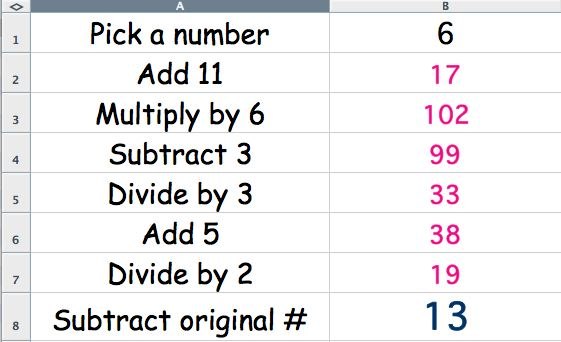Lesson: Jinx Puzzle
 Student Teacher Extensions
Here is a puzzle that may surprise you. Follow these steps:

Pick a number (1-10)  __________
Multiply by 6                __________
Subtract 3                    __________
Divide by 3                  __________
Divide by 2                  __________
Subtract the
original number           __________

Does this puzzle always work? (That is, are you always jinxed with the number 13?)
Try it again with 3 different numbers. (They don't have to be between 1 and 10.)

 First number Second number Third number Pick a number Add 11 Multiply by 6 Subtract 3 Divide by 3 Add 5 Divide by 2 Subtract the original number Your answer is:

Do you think you will always get 13 no matter what number you try?
Could you find a number that will unjinx the jinx puzzle?

The Jinx Calculator
To help you find out if this puzzle will work for all numbers, we will use a special spreadsheet “calculator."
Here's what your Jinx Calculator will look when you open it. Notice that 6 was the chosen number and entered in cell B1.The spreadsheet then quickly did all the calculations and the answer was once again 13 - the unlucky number. Can you find a number that will make the puzzle not end in 13 (and not jinx you?) Use the Excel version of the Jinx Calculator to help you.

(Hint: Try different kinds of numbers not just whole numbers.  Like these: 2.5, -56, 3000, 999999999, .000005. Fractions can also be tried. For example if you try 1/2, you enter =1/2 into the cell.

Share a few of your interesting examples of numbers that you used here.

Go to the results page and describe the results.

Questions: